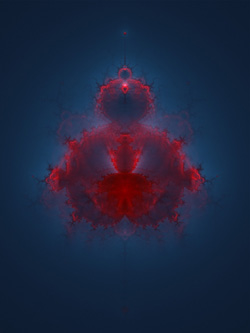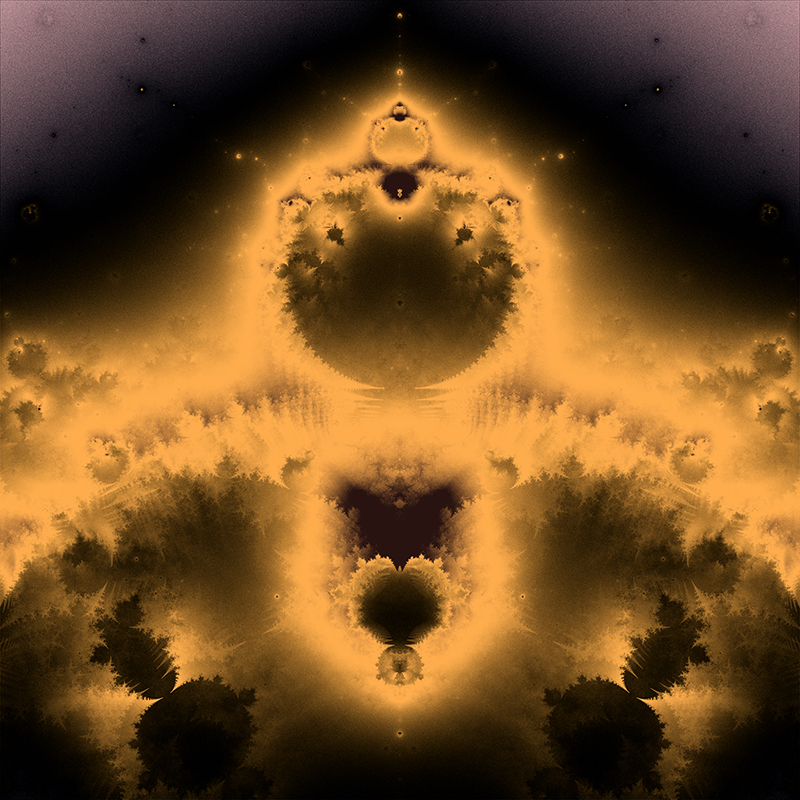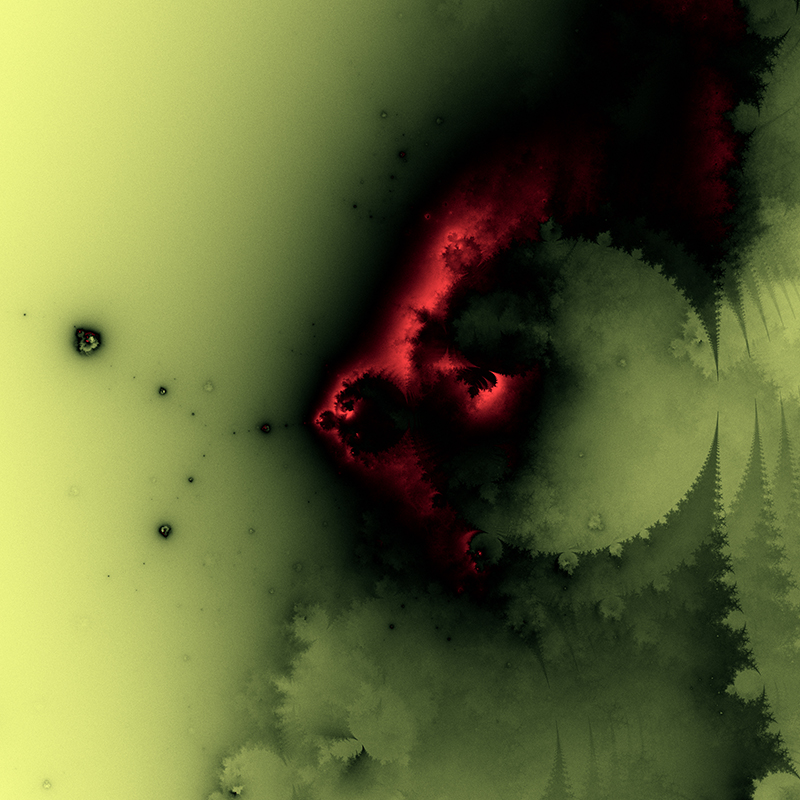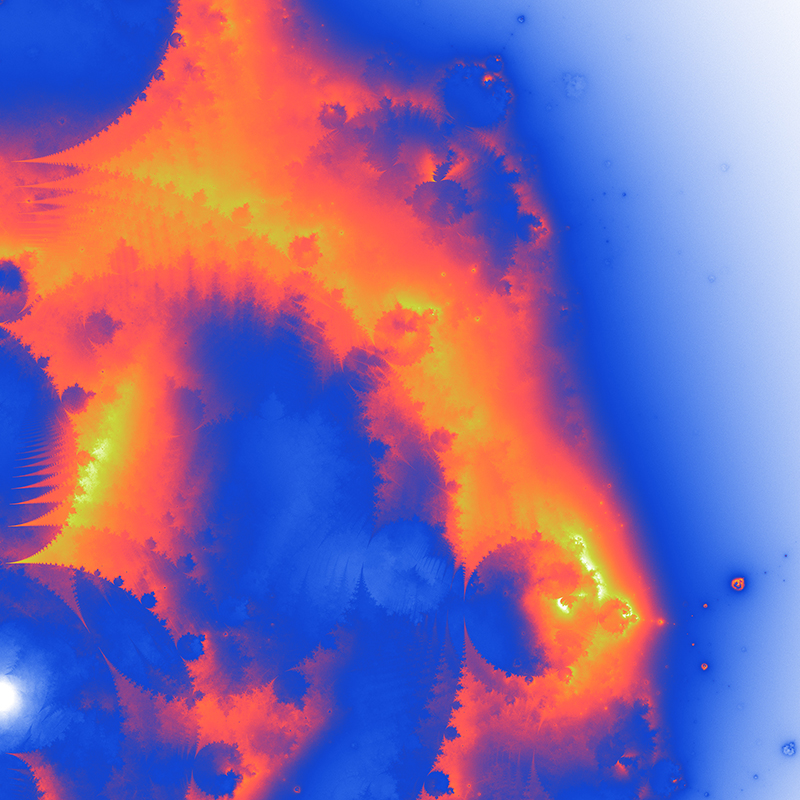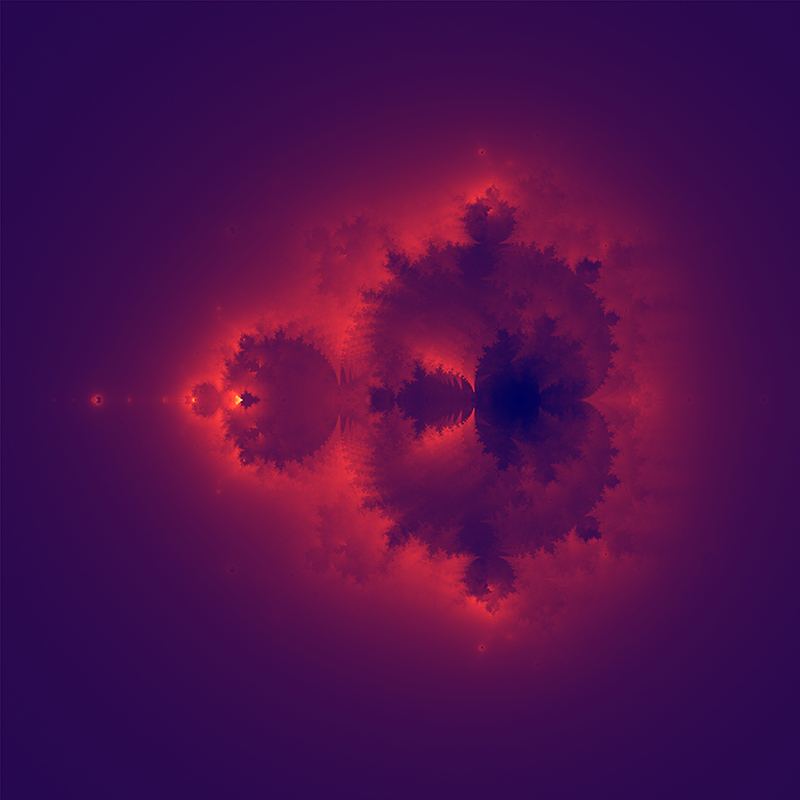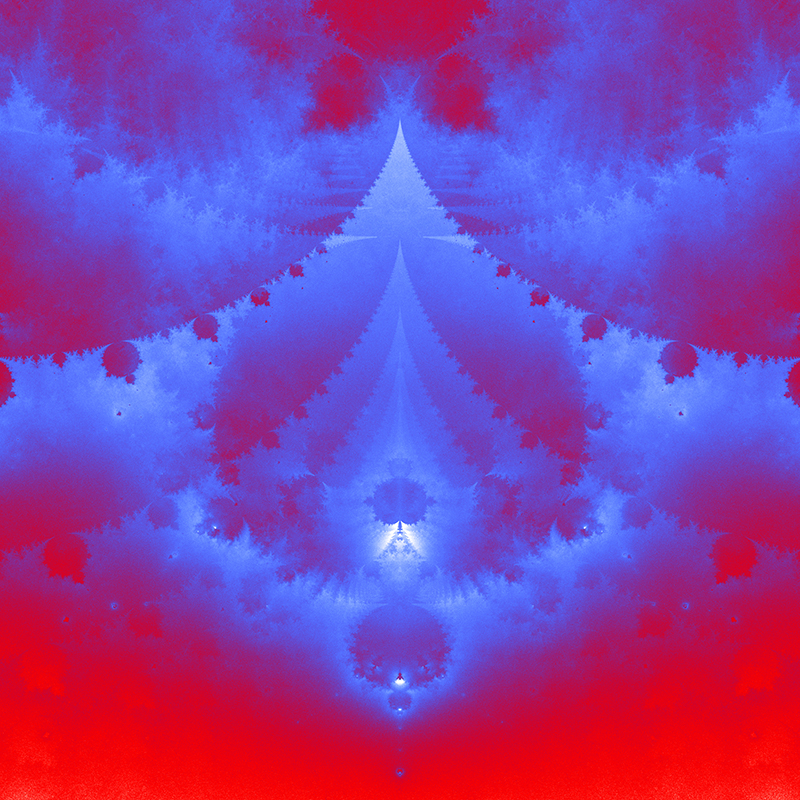# The Buddhabrot

Written by Paul Bourke
November 2000

Inspired by the Mandelbrot rendering technique attributed to Linas Vepstas
Explored by Melinda Green and Lori Gardi who coined the name.

The images on this page are created using the standard iterative series of the Mandelbrot, that is, iterate the function zn+1 = zn2 + z0 where z0 is each point in the image plane (complex plane). However, instead of recording the behavior of the series at each point z0 we now consider only those points that escape to infinity and we create a density plot of the terms in the series. The result then is a 2D density plot of the trajectories that escape to infinity. The following shows the buddhabrot for that part of the complex plane that is interesting.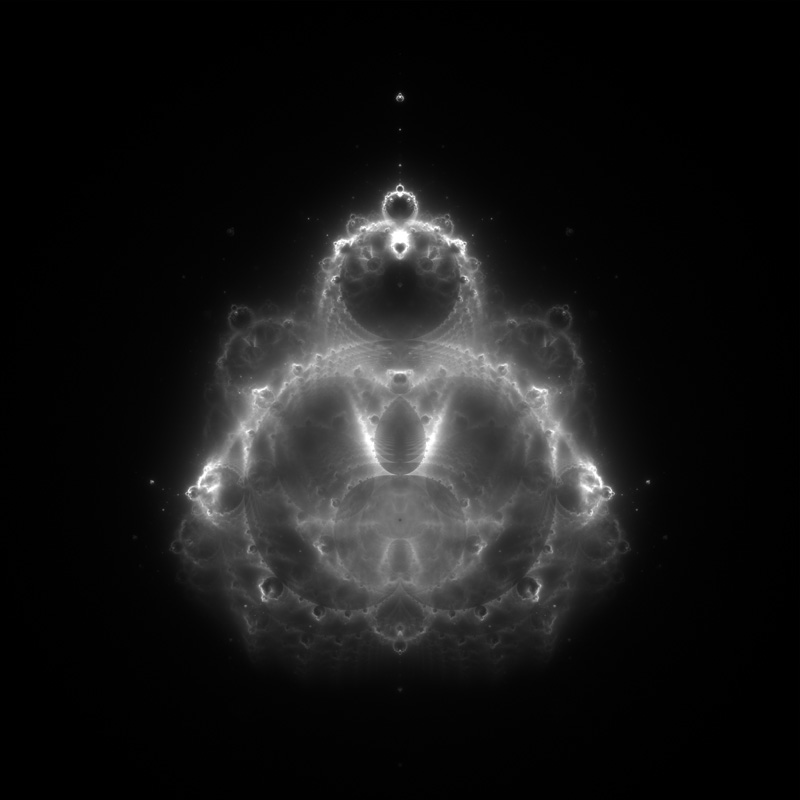Complex plane: -13/6 <= real <= 7/6 and -5/3 <= imaginary <= 5/3

In the following, the boundary of the Mandelbrot is shown in the same coordinate system in yellow. Note that images here have the imaginary axis horizontal and the real axis vertically, this is a 90 degree clockwise rotation from the way the Mandelbrot is traditionally drawn.One unfortunate consequence of how these images are created is that zooming in becomes increasingly inefficient. Points on an escape trajectory that pass through an arbitrary small region of the complex plane could have come from distant regions. So when computing the buddhabrot of a zoomed in portion of the complex plane, one still needs to choose z0 values from the whole plane. This isn't quite true, since all points outside the circle of radius 2 escape monotonically one only has to sample points to the region around the origin. As the area being computed gets smaller the probability of a trajectory passing through it deceases so it takes more iterations before the density plot becomes significant.While the normal Mandelbrot is "dull" on the interior, the buddhabrot has lots of internal structure.Unlike the Mandelbrot where choosing the number of iterations that are tested in order to determine whether the point escapes or not (often called the "bail-out") doesn't have a profound effect on the actual structure, in the buddhabrot it can. For example in the following the first uses a bail-out of 200 and the second uses 20. For very high quality versions of the buddhabrot a bail-out above 1000 is suggested, of course that increases compute times.Bail-out: 200Bail-out: 20

There are a number of ways one might colour the buddhabrot, the most common is to compute the buddhabrot a number of times with different number of iterations. Each of these images is mapped to a colour and combined. One can similarly combine images with different bail-out values (right), a common technique in astrophotography for combining images of different "exposures".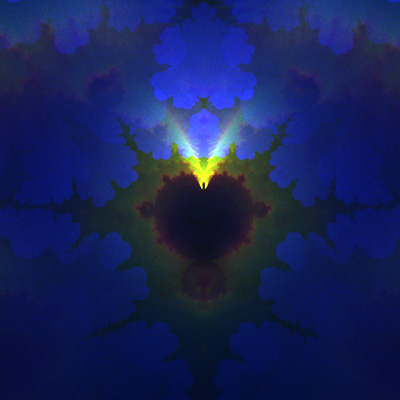The soul of the Buddha